# P Chart with JMP

### What is a P Chart?

The P chart plots the percentage of defectives in one subgroup as a data point. It considers the situation when the subgroup size of inspected units is not constant. The underlying distribution of the P-chart is binomial distribution.

### P Chart Equations

Data Point: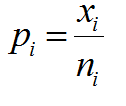Center Line: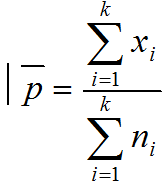Control Limits: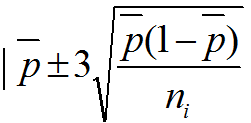Where:

• ni is the subgroup size for the ith subgroup
• k is the number of subgroups
• xi is the number of defects in the ith

### Use JMP to Plot a P Chart

Data File: “P.jmp”

1. Analyze -> Quality & Process -> Control Chart -> P
2. Select Fail in the Process Field
3. Select N in the Sample Size Field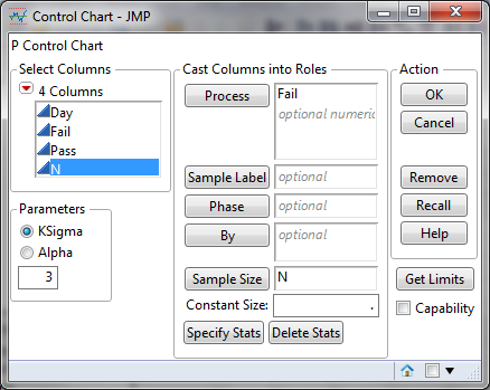4. Click Ok

#### P Chart Diagnosis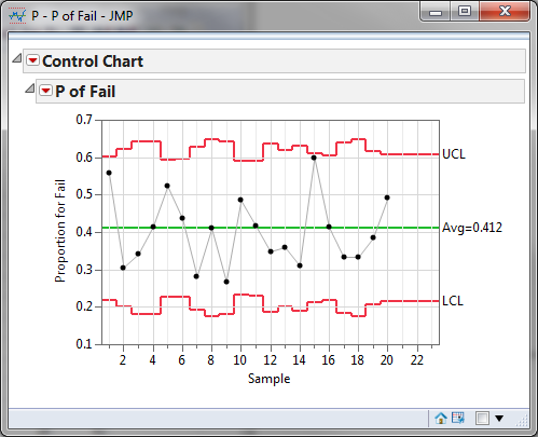Model summary: Since the sample sizes are not constant over time, the control limits are adjusted to different values accordingly. All the data points fall within the control limits and spread randomly around the mean. We conclude that the process is in control.